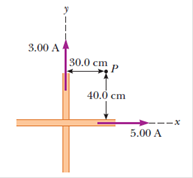Two long, straight wires cross each other at right angles, as shown in Figure P19.67. (a) Find the direction and magnitude of the magnetic field at point P, which is in the same plane as the two wires. (b) Find the magnetic field at a point 30.0 cm above the point of intersection (30.0 cm out of the page, toward you).FIGURE P19.67

Question

Two long, straight wires cross each other at right angles, as shown in Figure P19.67. (a) Find the direction and magnitude of the magnetic field at point P, which is in the same plane as the two wires. (b) Find the magnetic field at a point 30.0 cm above the point of intersection (30.0 cm out of the page, toward you).

FIGURE P19.67